> restart;

> f2:=x-> 2/Pi*(sin(x/2)): f3:=x-> 2/Pi*(sin(x/2)-sin(x)): f4:=x-> 2/Pi*(sin(x/2)-sin(x)+1/3*sin(3/2*x)):

> f5:= x -> Sum(2/(n*Pi)*(cos(n/2*Pi)-cos(n*Pi))*sin(n/2*x),n=1..5);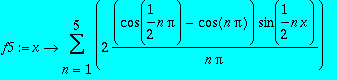> h:= x-> piecewise(-2*Pi<x and x<-Pi,-1,-Pi<x and x < Pi, 0, Pi<x and x< 2*Pi, 1);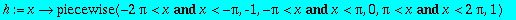> plot(h(x), x=-2*Pi..2*Pi, title="Example 3: jump");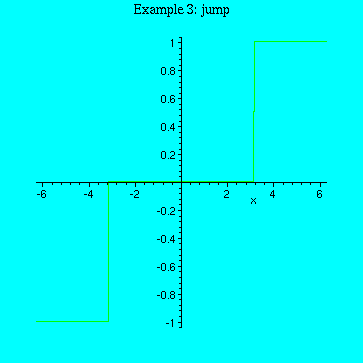> plot([h(x),f2(x), f3(x), f4(x)], x=-2*Pi..2*Pi, title="Ex 3, approximations 1,2,3", color=[black,black,black,black]);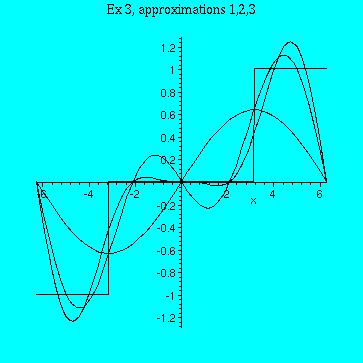> plot([h(x), f5(x)], x=-2*Pi..2*Pi, title = "Ex 3, fifth order approximation", color=[black,black]);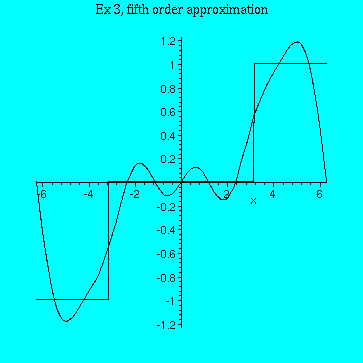> f25:= x -> Sum(2/(n*Pi)*(cos(n/2*Pi)-cos(n*Pi))*sin(n/2*x),n=1..25):

> plot([h(x), f25(x)], x=-2*Pi..2*Pi, title = "Ex 3, 25-th order approximation", color=[black,black]);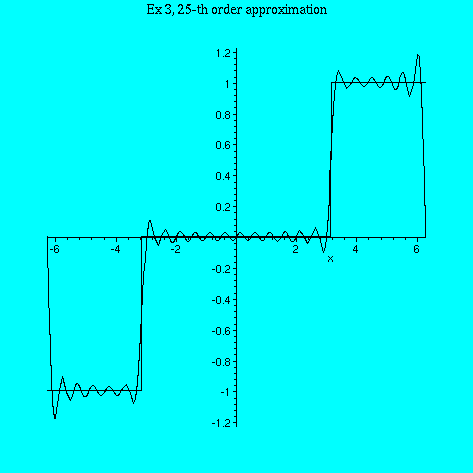> f50:= x -> Sum(2/(n*Pi)*(cos(n/2*Pi)-cos(n*Pi))*sin(n/2*x),n=1..50):

> plot([h(x), f50(x)], x=-2*Pi..2*Pi, title = "Ex 3, 50-th order approximation", color=[black,black]);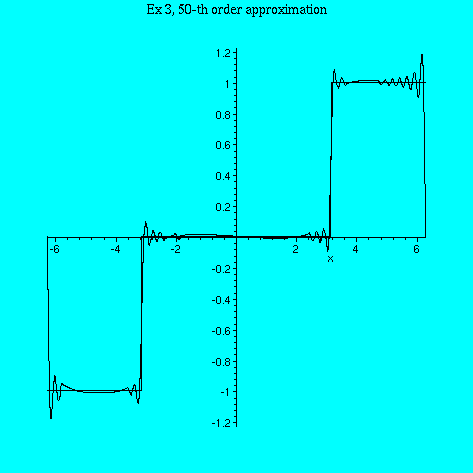> f500:= x -> Sum(2/(n*Pi)*(cos(n/2*Pi)-cos(n*Pi))*sin(n/2*x),n=1..500):

> plot([h(x), f500(x)], x=-2*Pi..2*Pi, title = "Ex 3, 500-th order approximation", color=[black,black]);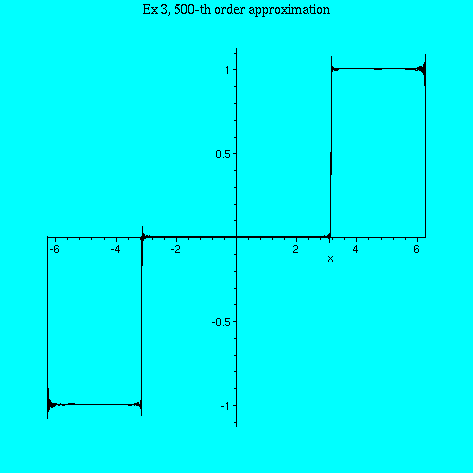> plot([h(x), f500(x)], x=-6*Pi..6*Pi, title = "Ex 3, 500-th order approximation, periodic extension", color=[black,black]);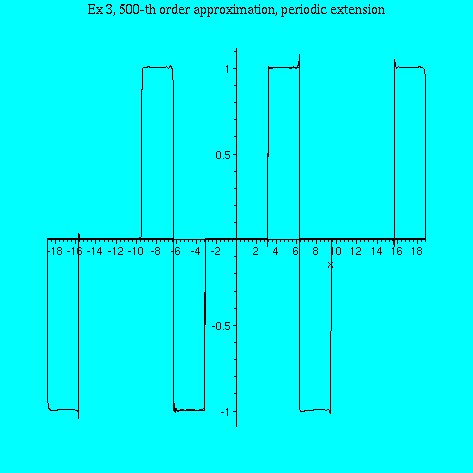>